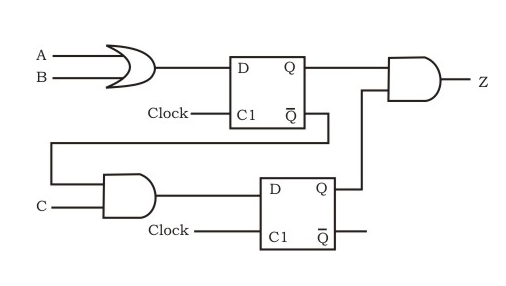# GATE | Gate IT 2005 | Question 43

Which of the following input sequences will always generate a 1 at the output z at the end of the third cycle?(A) 0 0 0
1 0 1
1 1 1
(B) 1 0 1
1 1 0
1 1 1
(C) 0 1 1
1 0 1
1 1 1
(D) 0 0 1
1 1 0
1 1 1

Explanation:

```Let's take cycles as 1,2,3.

There are two flip-flops, let's call them D1 and D2.
And Q1, Q2 to be the output for flip-flop D1, D2 respectively.
here Q11 means output of D1 flip-flop for 1st cycle, similarly Q12
is the output of D1 flip-flop for 2nd Cycle.
A1, A2, A3 means input for A at cycles 1, 2, 3 respectively. Similarly for B and C.

Let's check for the option D where:

A1=0 A2=1 A3=1
B1=0 B2=1 B3=1
C1=1 C2=0 C3=1

Q11=0
Q12 = A1.B1 = 0.0 = 0
Q13 = A2.B2 = 1.1 = 1
~Q11=1
~Q12 = ~(A1.B1) = 1
~Q13 = ~(A2.B2) = 0
Q21 = 0
Q22 = C1.(~Q11) = 1.1 = 1
Q23 = C2.(~Q12) = 0.1 = 0

Z1 = 0
Z2 = Q12.Q21 = 0.0 = 0
Z3 = Q13.Q22 = 1.1 = 1

Hence, sequence given in option D is generating a 1 at the output z at the
end of the third cycle (Z3).
```

This explanation has been contributed by Harshit Sidhwa.

Quiz of this Question

My Personal Notes arrow_drop_up
Article Tags :

Be the First to upvote.

Please write to us at contribute@geeksforgeeks.org to report any issue with the above content.